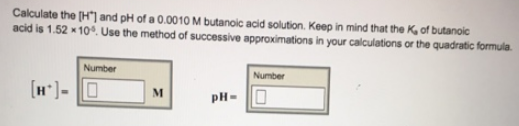# Problem: Calculate the [H+] and pH of a 0.0010 M butanoic acid solution. Keep in mind that the Ka of butanoic acid is 1.52 x 10-6. Use the method of successive approximations in your calculations or the quadratic formula.

###### FREE Expert Solution
95% (414 ratings)###### Problem Details

Calculate the [H+] and pH of a 0.0010 M butanoic acid solution. Keep in mind that the Ka of butanoic acid is 1.52 x 10-6. Use the method of successive approximations in your calculations or the quadratic formula.